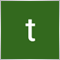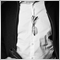# Problem with MathRand() in StrategyTester20

Hi,I'm new to programing.

I have problems using the Mathrand() function in the strategy tester.

I hope to conduct a MONKEY TEST with the Mathrand() function, but it does not operate in a way I want.

In a Monkey Test, I hope to create a series of random trade results, by Entering / Exiting each trades depending on the value generated from MathRand.

In addition, my aim here is to conduct the MONKEY TEST 10000 times ; therefore by setting 'Repeat_value' as an input parameter and optimizing this ( 1-10000, step:1 ).

However, the result here is that every  10 to 20 optimization result turn out to be the same.(The only one result without optimizing is all different and seems good.)

```//Exit rules

int shift = 0;

OrderSelect(LatestTicket,SELECT_BY_TICKET);

datetime myopentime = OrderOpenTime();

shift = iBarShift(NULL,PERIOD_CURRENT,myopentime,True);

bool EXIT_LONG_CONDITION  = false;

bool EXIT_SHORT_CONDITION = false;

int shiftnum = MathRand()%(2* holdbars);

if (shift > shiftnum)        //when to exit LONG

{

//Print("shiftnum = ",shiftnum);

EXIT_LONG_CONDITION = true;

}

if (shift >  MathRand()%(15* holdbars))        //when to exit SHORT

{

//Print("shiftnum = ",shiftnum);

EXIT_SHORT_CONDITION = true;

}

//Entry rules

int num1000 = MathRand()%1000;

int num100 = MathRand()%100;

//Print("num100 = ",num100);

//Print("num1000 =",num1000);

if (num100 <= longposition && possibilityoftrades >= num1000)  LONGCONDITION  = true;

if (num100 > longposition && possibilityoftrades >= num1000)  SHORTCONDITION = true;
```218606

tommy Y:

Hi,I'm new to programing.

I have problems using the Mathrand() function in the strategy tester.

I hope to conduct a MONKEY TEST with the Mathrand() function, but it does not operate in a way I want.

In a Monkey Test, I hope to create a series of random trade results, by Entering / Exiting each trades depending on the value generated from MathRand.

In addition, my aim here is to conduct the MONKEY TEST 10000 times ; therefore by setting 'Repeat_value' as an input parameter and optimizing this ( 1-10000, step:1 ).

However, the result here is that every  10 to 20 optimization result turn out to be the same.(The only one result without optimizing is all different and seems good.)

//Exit rules

int shift = 0;

OrderSelect(LatestTicket,SELECT_BY_TICKET);

datetime myopentime = OrderOpenTime();

shift = iBarShift(NULL,PERIOD_CURRENT,myopentime,True);

bool EXIT_LONG_CONDITION  = false;

bool EXIT_SHORT_CONDITION = false;

int shiftnum = MathRand()%(2* holdbars);

if (shift > shiftnum)        //when to exit LONG

{

//Print("shiftnum = ",shiftnum);

EXIT_LONG_CONDITION = true;

}

if (shift >  MathRand()%(15* holdbars))        //when to exit SHORT

{

//Print("shiftnum = ",shiftnum);

EXIT_SHORT_CONDITION = true;

}

//Entry rules

int num1000 = MathRand()%1000;

int num100 = MathRand()%100;

//Print("num100 = ",num100);

//Print("num1000 =",num1000);

if (num100 <= longposition && possibilityoftrades >= num1000)  LONGCONDITION  = true;

if (num100 > longposition && possibilityoftrades >= num1000)  SHORTCONDITION = true;

Use MathSrand() before a call to MathRand()Documentation on MQL5: Math Functions / MathSrand
• www.mql5.com
MathSrand - Math Functions - MQL5 Reference - Reference on algorithmic/automated trading language for MetaTrader 520

Use MathSrand() before a call to MathRand()

I tried different parameterss for MathSrand() for each optimization, but some results remain to be the same.

What parameter should I input in MathSrand() if used in the strategy tester?

Or is there another problem with my coding of random number?24772

1. Please edit your (original) post and use the CODE button (Alt-S)! (For large amounts of code, attach it.)
General rules and best pratices of the Forum. - General - MQL5 programming forum 2019.05.06
Messages Editor

2. `       OrderSelect(LatestTicket,SELECT_BY_TICKET);  `
You don't test if the ticket has already been closed.20

William Roeder:
1. Please edit your (original) post and use the CODE button (Alt-S)! (For large amounts of code, attach it.)
General rules and best pratices of the Forum. - General - MQL5 programming forum 2019.05.06
Messages Editor

2. You don't test if the ticket has already been closed.

Sorry,I edited.

Sorry, I omitted some parts of the original code -

well first of all I gained 'the current market position' by using OrderSelect(), and executed market orders if only 'LONG/SHORT conditions' and conditions based on 'the current market position' ( ex. go long only when your market position is 0 or short) were both met.4227

Try this:

```//--- Initialize the generator of random numbers
MathSrand(GetTickCount()^(uint)ChartID());```20

amrali:

Try this: